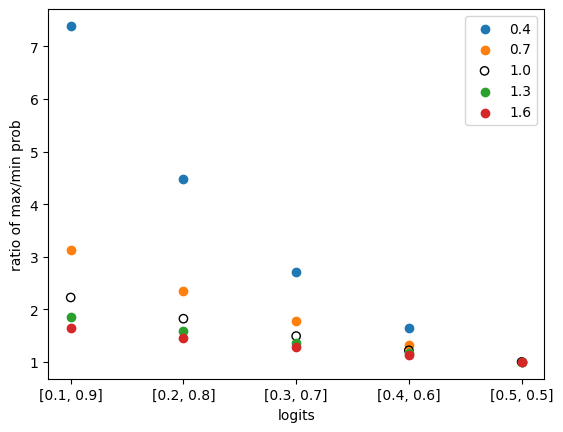TL:DR OpenAI 的 ChatGPT 在其官方文档（https://platform.openai.com/docs/api-reference/chat/create）中给出了各种参数的范围和含义。我们将讨论 ChatGPT 的生成过程和这些参数是如何实现其生成的效果的。

## ChatGPT 的解码过程#

1. 将用户的请求，从 1 个扩充成 num_samples 大小的 batch
2. 进行模型推理，得到 logits
3. 进行 temperature 映射：logits = logits / temperature
4. [可选] 进行 topk 处理：logits = topk_func(logits, top_k)
5. logits 到 概率的转换：probs = softmax(logits)
6. 是否 sample：
1. 进行 sample：idx_next = multinomial_sample(probs, num_samples=1)
2. 不进行 sample：idx_next = topk_func(probs, k=1)
7. 重复上述过程 max_new_tokens 次

## ChatGPT 的解码参数#

### temperature#

temperature 参数的官方定义如下：

temperature number Optional Defaults to 1

What sampling temperature to use, between 0 and 2. Higher values like 0.8 will make the output more random, while lower values like 0.2 will make it more focused and deterministic.

We generally recommend altering this or `top_p` but not both.

1. 假设某个模型的 vocabulary 的大小为 2，在某个时刻，模型的输出为 logits = [0.8, 0.2]。
2. 如果不进行 temperature 映射（等价于将 temperature 设置为 1， 也就是默认值）： 概率转换：probs = softmax(logits) = [0.65, 0.35]
3. 如果 temperature 设置为 1.8，那么 logits = logits / temperature = [0.44, 0.11], 下一步进行概率转换：probs = softmax(logits) = [0.58, 0.42]
4. 如果 temperature 设置为 0.2，那么 logits = logits / temperature = [4, 1], 下一步进行概率转换：probs = softmax(logits) = [0.95, 0.05]

``````# importing package
import matplotlib.pyplot as plt
import numpy as np
import math

# x axis index and values
data = list(enumerate(zip(np.arange(0.1, 0.6, 0.1), np.arange(0.9, 0.4, -0.1))))

# colors for each temperature, from low to high temperature, from yellow to dark red
# reference: https://colorbrewer2.org/#type=sequential&scheme=YlOrRd&n=5
colors = ["#ffffb2", "#fecc5c", "#fd8d3c", "#f03b20", "#bd0026"]

for t_idx, temperature in enumerate(np.arange(0.4, 1.6 + 0.0001, 0.3)):
# each line for each temperature

# get x and y values
x = []
y = []
for x_idx, (a, b) in data:
logits = np.array([a, b])
probs = softmax(logits / temperature)
x.append(x_idx)
y.append(probs / probs) # max prob / min prob

# plot
circle_color = colors[t_idx]
if math.isclose(temperature, 1.0):
# plot the line for temperature 1.0 with black circles
plt.scatter(x, y, label=f"{temperature:.1f}", facecolors="black", edgecolors="black")
else:
# other lines with colorful lines
plt.scatter(x, y, label=f"{temperature:.1f}", facecolors=circle_color, edgecolors="gray")

plt.legend()

# set x and y axis
plt.xlabel('logits')
plt.xticks([x for x, _ in data],
[f"[{a:.1f}, {b:.1f}]" for _, (a, b) in data])
plt.ylabel('ratio of max/min prob')

plt.show()
``````### top_p#

top_p 参数的官方定义如下：

top_p number Optional Defaults to 1

An alternative to sampling with temperature, called nucleus sampling, where the model considers the results of the tokens with top_p probability mass. So 0.1 means only the tokens comprising the top 10% probability mass are considered.

We generally recommend altering this or `temperature` but not both.

### stop#

stop 参数的官方定义如下：

stop string or array Optional Defaults to null

Up to 4 sequences where the API will stop generating further tokens.

### n#

n 参数的官方定义如下：

n integer Optional Defaults to 1

How many chat completion choices to generate for each input message.

### max_tokens#

max_tokens 参数的官方定义如下：

max_tokens integer Optional Defaults to inf

The maximum number of tokens to generate in the chat completion.

The total length of input tokens and generated tokens is limited by the model’s context length.

• stop 参数的设置，详情请见上文。
• 可能的特殊的休止符 token。通过实际使用 ChatGPT，可以发现 ChatGPT 并不会机械的输出指定的文本长度，在充分回答问题后，就会自行停止。
• 实验代码如下：
``````import openai

openai.api_key = "sk-xxx"

completion = openai.ChatCompletion.create(
model="gpt-4",
messages=[{"role": "user", "content": "你帮我输出1到10之间的偶数，输出时，每个数字之间用一个空格隔开。除了数字，其他的都不要输出。"}],
temperature=0,
max_tokens=100,
)

response = completion.choices.message["content"]
print("length: ", len(response))  # 将会输出：length:  10
print(response)  # 将会输出：2 4 6 8 10
``````

### presence_penalty#

presence_penalty 参数的官方定义如下：

frequency_penalty number Optional Defaults to 0

Number between -2.0 and 2.0. Positive values penalize new tokens based on their existing frequency in the text so far, decreasing the model’s likelihood to repeat the same line verbatim.

presence_penalty 名字中虽然带着 penalty，但由于其取值范围可能是正数也可能是负数，因此并不一定是惩罚 token 的反复出现，也有可能是鼓励反复出现。

### frequency_penalty#

frequency_penalty 参数的官方定义如下：

frequency_penalty number Optional Defaults to 0

Number between -2.0 and 2.0. Positive values penalize new tokens based on their existing frequency in the text so far, decreasing the model’s likelihood to repeat the same line verbatim.

### logit_bias#

logit_bias 参数的官方定义如下：

logit_bias map Optional Defaults to null

Modify the likelihood of specified tokens appearing in the completion.

Accepts a json object that maps tokens (specified by their token ID in the tokenizer) to an associated bias value from -100 to 100. Mathematically, the bias is added to the logits generated by the model prior to sampling. The exact effect will vary per model, but values between -1 and 1 should decrease or increase likelihood of selection; values like -100 or 100 should result in a ban or exclusive selection of the relevant token.